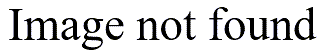# Randomization test

## 2004-07-14

I received some data from a project where the outcome measure was the degree of improvement after a treatment, with values of -1 (slight decline), 0 (no change), 1 (slight improvement), 2 (moderate improvement), and 3 (large improvement). The two treatments had quite different results. The old therapy had eight patients, three of whom showed a slight decline and five of whom showed no change. Among the eight patients in the new therapy, one showed no change, three showed a slight improvement, six showed moderate improvement, and two showed a large improvement. There are several approaches that you could try with this data.

Some people might consider this ordinal data and would discourage the computation of averages. I didn’t see this as a serious issue because computing an average of ordinal data never seemed like a terrible thing to me. After all, my Grade Point Average from college is clearly an average of ordinal data with A=4, B=3, C=2, D=1, and F=0.

So the first thing to try is a simple t-test. Here’s how you would enter the data and run a t-test in S-plus or R.

old <- rep(-1:0,c(3,5)) new <- rep(0:3,c(1,3,6,2)) old  -1 -1 -1 0 0 0 0 0 new  0 1 1 1 2 2 2 2 2 2 3 3 mean(old)  -0.375 mean(new)  1.75 t.test(old,new) Standard Two-Sample t-Test data: old and new t = -6.2075, df = 18, p-value = 0 alternative hypothesis: difference in means is not equal to 0 95 percent confidence interval: -2.844201 -1.405799 sample estimates: mean of x mean of y -0.375 1.75

Even though I did not have a problem with computing averages, I was a bit nervous about the t-test. This data is clearly non-normal, and with the sample sizes as small as they are, I’d be worried about whether the t-test would be valid. An interesting alternative is the randomization test.

The randomization test is a very useful test, but I had never had a chance to run one before. The concept is quite intriguing. The difference between the two groups is 2.125 (1.75 - (-0.375)) but you are unsure how much sampling error there is in this estimate. You don’t want to rely on the traditional formula for standard error. So you take the twenty value and mix them up randomly and compute a new average difference.

What a second, if you mix up the data and recompute a mean difference, isn’t what you are getting pure noise? Precisely, and if we do this repeatedly, we will get a good handle on how noisy this data is.

Here’s an Excel sheet that shows the original data and 25 random shuffles of the data.Notice that the worst case among the 25 permutations was only 1.29 which is well short of the value you observed, 2.125. So this gives you some idea that the difference you actually observed is probably well beyond what you might expect from sampling error. You can formalize this by randomizing the data a few hundred or a few thousand times, and noting how often (or how rarely) the randomized statistic exceeded the observed statistic. If this is small (say less than 5% of the time), then you would conclude that the two groups are not comparable.

Randomization tests are very useful for microarray research because these experiments usually have a very small sample size which makes it difficult to use traditional tests.

Phillip Good is a strong proponent for the use of randomization tests. His book, Resampling Methods. A Practical Guide to Data Analysis [BookFinder4U link]](http://www.bookfinder4u.com/detail/0817642439.html), is an excellent introduction to this area.